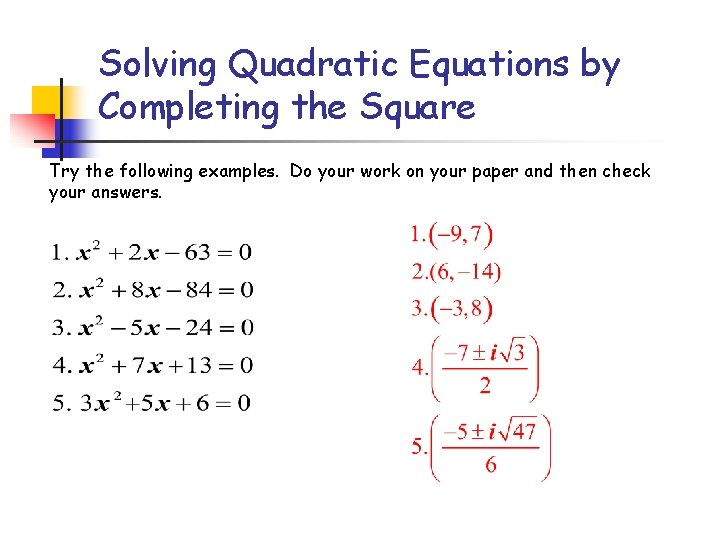# Solving Quadratic Equations by Completing the Square Perfect

• Slides: 14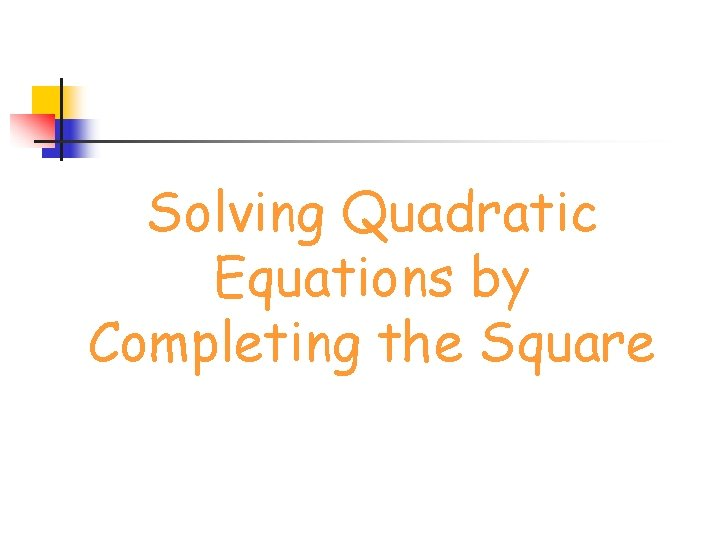Solving Quadratic Equations by Completing the SquarePerfect Square Trinomials Examples l x 2 + 6 x + 9 l x 2 - 10 x + 25 l x 2 + 12 x + 36 lCreating a Perfect Square Trinomial In the following perfect square trinomial, the constant term is missing. X 2 + 14 x + ____ l Find the constant term by squaring half the coefficient of the linear term. l (14/2)2 X 2 + 14 x + 49 l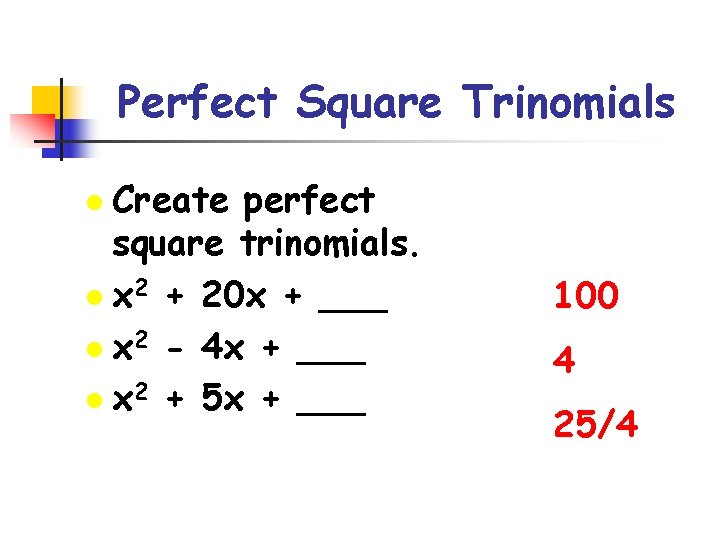Perfect Square Trinomials Create perfect square trinomials. l x 2 + 20 x + ___ l x 2 - 4 x + ___ l x 2 + 5 x + ___ l 100 4 25/4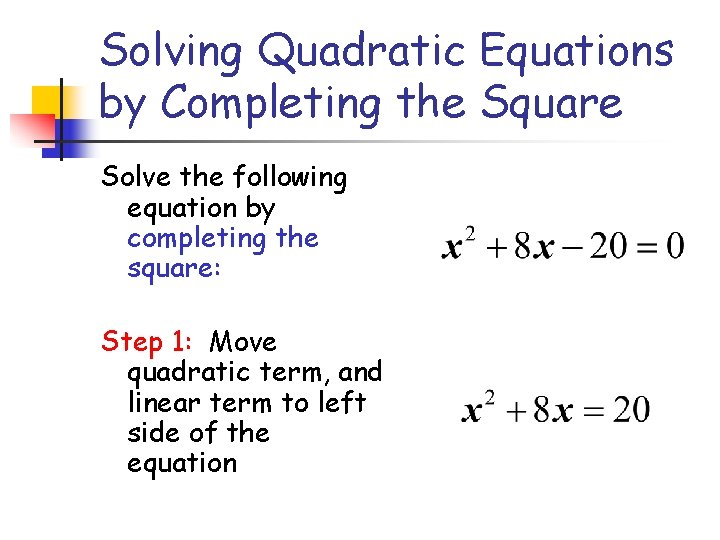Solving Quadratic Equations by Completing the Square Solve the following equation by completing the square: Step 1: Move quadratic term, and linear term to left side of the equationSolving Quadratic Equations by Completing the Square Step 2: Find the term that completes the square on the left side of the equation. Add that term to both sides.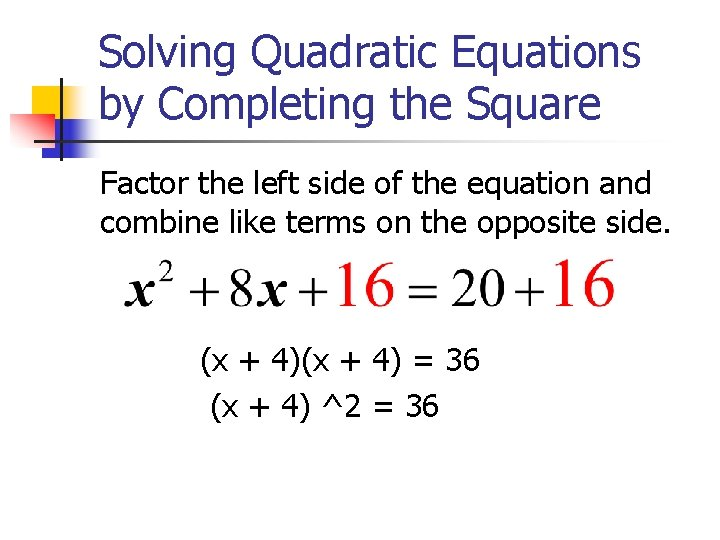Solving Quadratic Equations by Completing the Square Factor the left side of the equation and combine like terms on the opposite side. (x + 4) = 36 (x + 4) ^2 = 36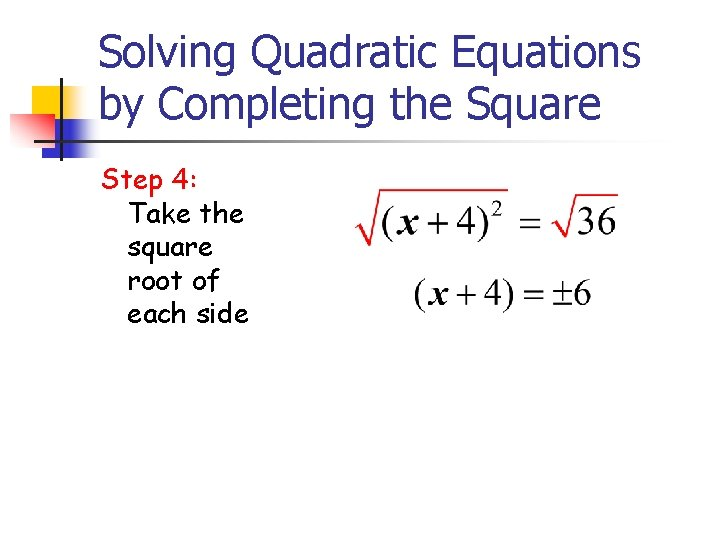Solving Quadratic Equations by Completing the Square Step 4: Take the square root of each side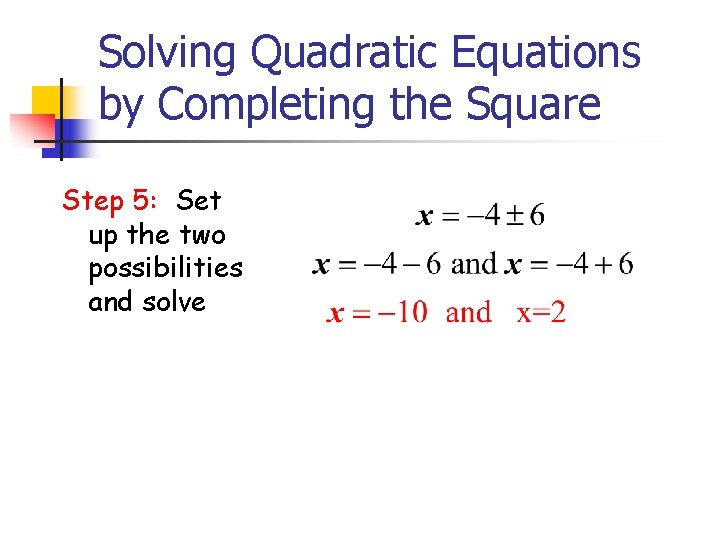Solving Quadratic Equations by Completing the Square Step 5: Set up the two possibilities and solveCompleting the Square-Example #2 Solve the following equation by completing the square: Step 1: Move quadratic term, and linear term to left side of the equation, the constant to the right side of the equation.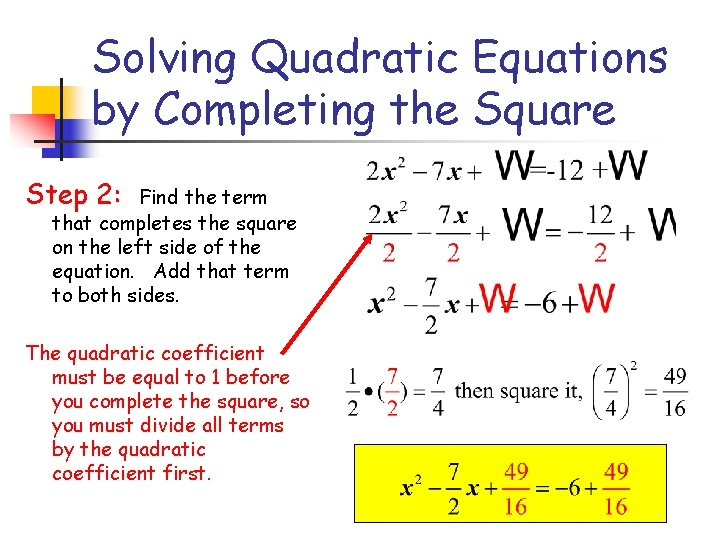Solving Quadratic Equations by Completing the Square Step 2: Find the term that completes the square on the left side of the equation. Add that term to both sides. The quadratic coefficient must be equal to 1 before you complete the square, so you must divide all terms by the quadratic coefficient first.Solving Quadratic Equations by Completing the Square Step 3: Factor the perfect square trinomial on the left side of the equation. Simplify the right side of the equation.Solving Quadratic Equations by Completing the Square Step 4: Take the square root of each side